# Rounding to the Nearest 10th

When working on word problems, we are often asked to round to the nearest tenth. This can make a really long, and possibly challenging answer easier to work with.

In order to round to the nearest tenth, we need to know where the tenths place is. Here is a visual look at place value: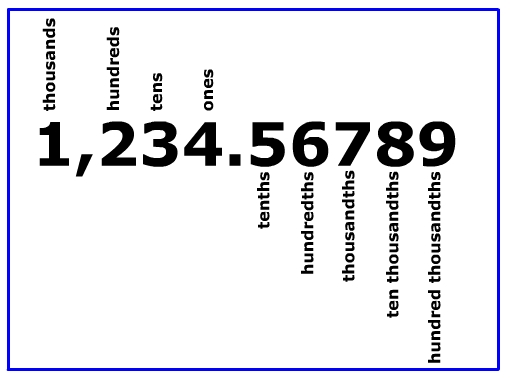The tenths place is to the right of the decimal point. Our rounded answer will stop at the tenths place.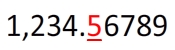We use the hundredths place to help us determine the value that needs to be in the tenths place.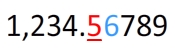If the value in the hundredths place is 5 or above "we give it a shove."

If the value is four or below, "we let it go."

In this example, the number is 6, which is "5 or above." So we give it a shove. This means that we round it up from 5 to 6.

Is 0.56 closer to 0.50 or 0.60? We know that 56 is closer to 60, so 0.56 is closer to 0.60 or 0.6. Therefore, we round the 5 up to a 6.

Here are some more examples. Round each number to the nearest tenth.

#1.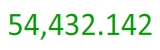Step 1: Locate the tenths place.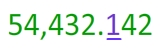Step 2: Look to the right of the tenths place and use the number to determine if you will round up or stay the same.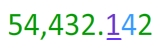Notice that the number to the right is less than 5. This means that the 1 will not round up to a 2. Instead, it will stay the same.

Step 3: Write the final answer that ends at the tenths place.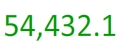#2.Step 1: Locate the tenths place.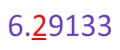Step 2: Look to the right of the tenths place and use the number to determine if you will round up or stay the same.Here, we have a 9 to the right of the tenths place. This is above 5, so we will round up from 2 to 3.

Step 3: Write the final answer that ends at the tenths place.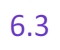#3.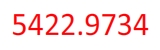Step 1: Locate the tenths place.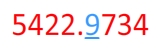Step 2: Look to the right of the tenths place and use the number to determine if you will round up or stay the same.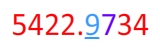This is an interesting example. The 7 tells us to round up. However, when we round up the nine becomes a ten. When this happens the nine becomes a zero and the place to the left is one bigger. Here is a look at the 9 becoming a 10. Notice that the 1 in the ten is under the next place value to the left.We can think of this as the 29 rounding up to a 30.

Step 3: Write the final answer that ends at the tenths place.Let's Review:

After you locate the tenths place, you will look to the right. If the number to the right is 5 or greater, you will round the tenths place up to the next digit.

If the number to the right is 4 or less, you will leave the tenths place alone.